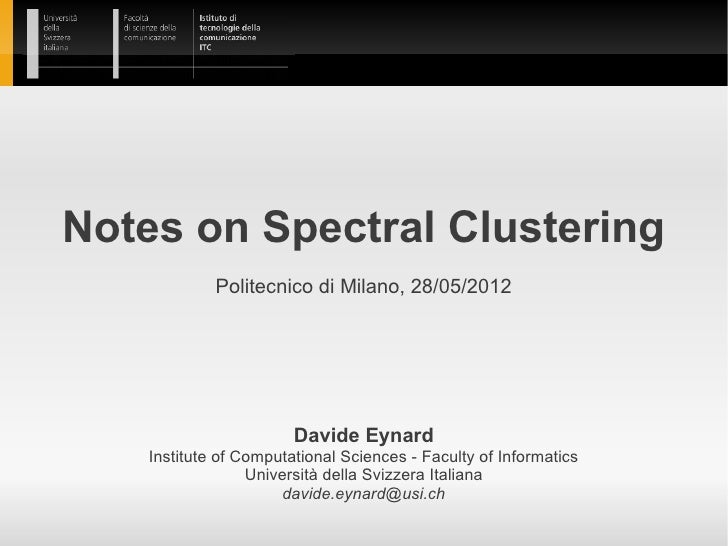Successfully reported this slideshow.
We use your LinkedIn profile and activity data to personalize ads and to show you more relevant ads. You can change your ad preferences anytime.Upcoming SlideShare
Loading in …5
×

# Notes on Spectral Clustering

2,748 views

Published on

Notes taken while reading the paper "A Tutorial on Spectral Clustering" by Ulrike von Luxburg. Find original paper at http://www.informatik.uni-hamburg.de/ML/contents/people/luxburg/publications/Luxburg07_tutorial.pdf

Published in: Technology
• Full Name
Comment goes here.

Are you sure you want to Yes No
Your message goes here• Be the first to comment

### Notes on Spectral Clustering

1. 1. Notes on Spectral Clustering Politecnico di Milano, 28/05/2012 Davide Eynard Institute of Computational Sciences - Faculty of Informatics Università della Svizzera Italiana davide.eynard@usi.ch
2. 2. Talk outline  Spectral Clustering  Distances and similarity graphs  Graph Laplacians and their properties  Spectral clustering algorithms  SC under the hood24/04/2011 Notes on Spectral Clustering and Joint Diagonalization 2/15
3. 3. Similarity graph  The objective of a clustering algorithm is partitioning data into groups such that:  Points in the same group are similar  Points in different groups are dissimilar  Similarity graph G=(V,E) (undirected graph)  Vertices vi and vj are connected by a weighted edge if their similarity is above a given threshold  GOAL: find a partition of the graph such that:  edges within a group have high weights  edges across different groups have low weights24/04/2011 Notes on Spectral Clustering and Joint Diagonalization 3/15
4. 4. Weighted adjacency matrix  Let G(V,E) be an undirected graph with vertex set V={v1,...,vn}  Weighted adjacency matrix W=(wij )i,j=1,...,n  wij≥0 is the weight of the edge between vi and vj  wij=0 means that vi and vj are not connected by an edge  wij=wji  Degree of a vertex vi∈V: di=∑j=1..nwij  Degree matrix D=diag(d1,...,dn)24/04/2011 Notes on Spectral Clustering and Joint Diagonalization 4/15
5. 5. Different similarity graphs  ε-neighborhood  Connect all points whose pairwise distance is less than ε  k-nearest neighbors  if vi ∈ knn(vj) OR vj ∈ knn(vi)  if vi ∈ knn(vj) AND vj ∈ knn(vi) (mutual knn)  after connecting edges, use similarity as weight  fully connected  all points with similarity sij>0 are connected  To control neighborhoods to be local, use a similarity function like the Gaussian: s(xi,xj)=exp(-║xi-xj║2/(2σ2))24/04/2011 Notes on Spectral Clustering and Joint Diagonalization 5/15
6. 6. Graph Laplacians  Graph Laplacian:  L=D–W (symmetric and positive semi-definite)  Properties  Smallest eigenvalue λ1=0 with eigenvector = �  n non-negative, real-valued eigenvalues 0=λ1≤λ2≤...≤λn  the multiplicty k of the eigenvalue 0 of L equals the number of connected components A1,...,Ak in the graph24/04/2011 Notes on Spectral Clustering and Joint Diagonalization 6/15
7. 7. Spectral Clustering algorithm (1) Spectral Clustering algorithm Input: Similarity matrix S ∈ ℝn×n, number k of clusters to construct. 1. Construct a similarity graph as previously described. Let W be its weighted adjacency matrix. 2. Compute the unnormalized Laplacian L 3. Compute the first k eigenvectors u1,…,uk of L 4. Let U ∈ ℝn×k be the matrix containing the vectors u1,…,uk as columns 5. For i=1,...,n let yi ∈ ℝk be the vector corresponding to the i-th row of U 6. Cluster the points (yi)i=1,...,n in ℝk with the k-means algorithm into clusters C1,...,Ck. Output: Clusters A1,...,Ak with Ai= {j | yj ∈ Ci}.24/04/2011 Notes on Spectral Clustering and Joint Diagonalization 7/15
8. 8. Normalized Graph Laplacians  Normalized graph Laplacians  Symmetric: Lsym=D-1/2LD-1/2 = I-D-1/2WD-1/2  Random Walk: Lrw=D-1L=I-D-1W  Properties  λ is an eigenvalue of Lrw with eigenvector u iff λ is an eigenvalue of Lsym with eigenvector w=D1/2u  λ is an eigenvalue of Lrw with eigenvector u iff λ and u solve the generalized eigenproblem Lu=λDu24/04/2011 Notes on Spectral Clustering and Joint Diagonalization 8/15
9. 9. Normalized Graph Laplacians  Normalized graph Laplacians  Symmetric: Lsym=D-1/2LD-1/2 = I-D-1/2WD-1/2  Random Walk: Lrw=D-1L=I-D-1W  Properties (follow)  0 is an eigenvalue of Lrw with � as eigenvector, and an eigenvalue of Lsym with eigenvector D1/2�.  Lsym and Lrw are positive semi-definite and have n non- negative, real-valued eigenvalues 0=λ1≤λ2≤...≤λn  the multiplicty k of the eigenvalue 0 of both Lsym and Lrw equals the number of connected components A1,...,Ak24/04/2011 Notes on Spectral Clustering and Joint Diagonalization 9/15
10. 10. Spectral Clustering algorithm (2) Normalized Spectral Clustering Input: Similarity matrix S ∈ ℝn×n, number k of clusters to construct.  Lrw: 3. Compute the first k generalized eigenvectors u1,…,uk of the generalized eigenproblem Lu=λDu  Lsym: 2. Compute the normalized Laplacian Lsym 3. Compute the first k eigenvectors u1,…,uk of Lsym 4. normalize the eigenvectors Output: Clusters A1,...,Ak with Ai= {j | yj ∈ Ci}.24/04/2011 Notes on Spectral Clustering and Joint Diagonalization 10/15
11. 11. A spectral clustering example24/04/2011 Notes on Spectral Clustering and Joint Diagonalization 11/15
12. 12. Under the hood  0-eigenvalues in the ideal case  parameters are crucial:  k in k nearest neighbors  σ in Gaussian kernel  k (another one!) in k-means24/04/2011 Notes on Spectral Clustering and Joint Diagonalization 12/15
13. 13. Random Walk point of view  Random walk: stochastic process which randomly jumps from one vertex to another  Clustering: finding a partition such that a random walk stays long within a cluster and seldom jumps between clusters  Transition probability pij:=wij/di  Transition matrix: P = D-1W => Lrw= I - P  λ is an eigenvalue of Lrw with eigenvector u iff 1-λ is an eigenvalue of P with eigenvector u24/04/2011 Notes on Spectral Clustering and Joint Diagonalization 13/15
14. 14. References  Von Luxburg, U. (2007). A tutorial on spectral clustering. Statistics and Computing, 17(4), 395-416. Springer.24/04/2011 Notes on Spectral Clustering and Joint Diagonalization 14/15
15. 15. Thank you! Thanks for your attention! Questions?24/04/2011 Notes on Spectral Clustering and Joint Diagonalization 15/15